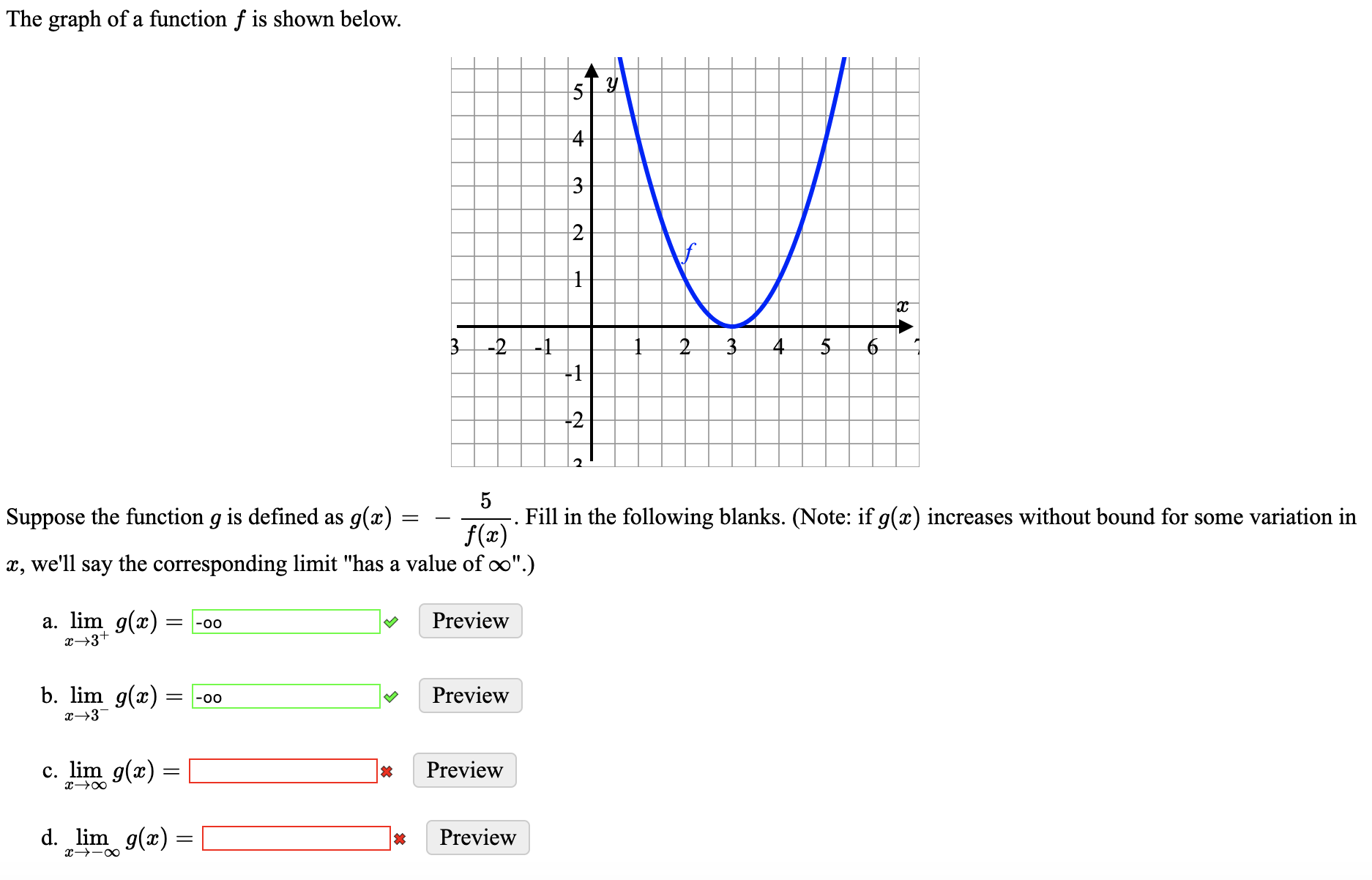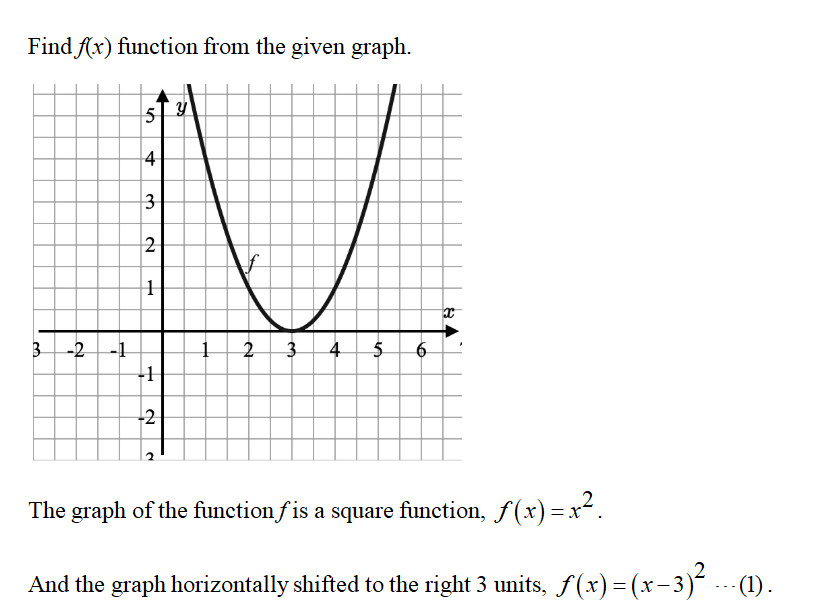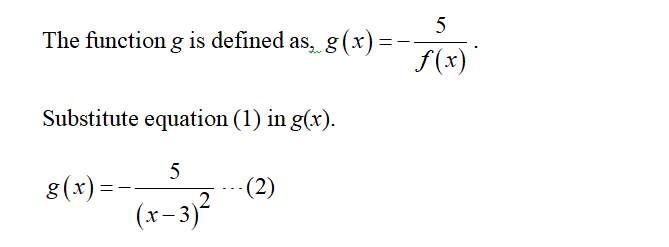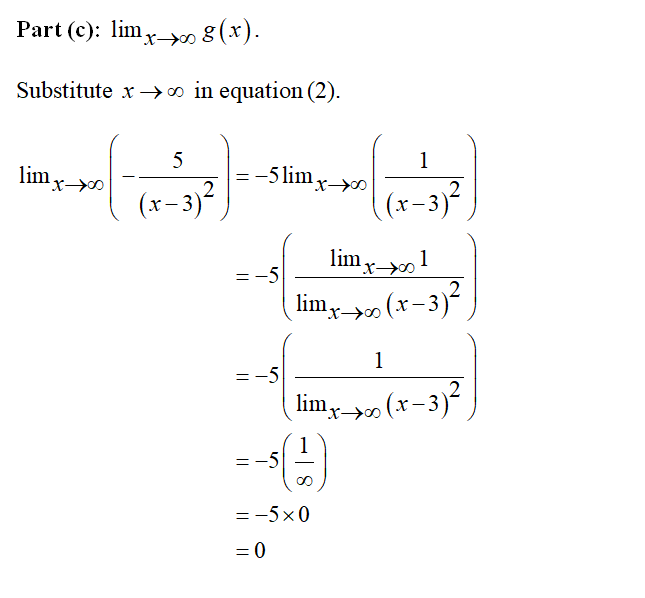# The graph of a function f is shown below.3-2 -1+1-25Fill in the following blanks. (Note: if g(x) increases without bound for some variation inSuppose the function g is defined as g(x)f(x)x, we'll say the corresponding limit "has a value of oo".)a. lim g(x) = -00х3+Previewb. lim g(x) = -00Previewг—3c. lim g(x) =Previewd. lim g(x) =Previewto4.4-

Question
53 viewshelp_outlineImage TranscriptioncloseThe graph of a function f is shown below. 3-2 -1 +1 -2 5 Fill in the following blanks. (Note: if g(x) increases without bound for some variation in Suppose the function g is defined as g(x) f(x) x, we'll say the corresponding limit "has a value of oo".) a. lim g(x) = -00 х3+ Preview b. lim g(x) = -00 Preview г—3 c. lim g(x) = Preview d. lim g(x) = Preview to 4. 4- fullscreen
check_circle

Step 1Step 2Step 3...

### Want to see the full answer?

See Solution

#### Want to see this answer and more?

Solutions are written by subject experts who are available 24/7. Questions are typically answered within 1 hour.*

See Solution
*Response times may vary by subject and question.
Tagged in

### Calculus